# SAT Math Multiple Choice Question 736: Answer and Explanation

### Test Information

Question: 736

1. If m is a number chosen randomly from the set {2, 3, 4, 6} and n is a number chosen randomly from the set {1, 2, 3, 4}, what is the probability that mn is a multiple of 12?

• A.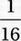• B.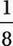• C.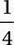• D.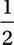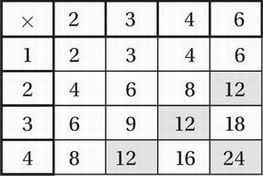This shows that 4 out of the possible 16 products are multiples of 12, and therefore, the probability is 4/16 or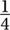.